Publication date: 05/24/2021

## Margin of Error for One Sample Mean

Use the Interval Explorer for one sample mean to determine a sample size for a confidence, prediction, or tolerance interval. Select DOE > Sample Size Explorers > Confidence Intervals > Margin of Error for One Sample Mean. Explore the trade offs between variability assumptions, sample size, significance, and the margin of error.

### Interval Explorer for One Sample Mean Options

Set study assumptions and explore sample sizes using the radio buttons, text boxes, and sliders. The curve updates as you make changes to the settings. Alternatively, change settings by dragging the cross hairs on the curve or adjusting the values in the axis text boxes.

Interval Purpose

Confidence

Specifies a confidence interval for a mean.

Prediction

Specifies a prediction interval for one future observation.

Tolerance

Specifies a tolerance interval to cover a proportion of the population.

Interval Type

Bound

Specifies a one-sided interval (upper or lower bound)

Interval

Specifies a two-sided interval.

Fixed Parameters

Alpha

Specifies the confidence level is 1 - alpha. The default alpha level is 0.05 for a 95% confidence interval.

Std Dev (σ)

Specifies the assumed population standard deviation.

Proportion

(Available only when Tolerance is selected for Interval Purpose.) Specifies the proportion of the population for the tolerance interval to cover.

Population standard deviation known

Specifies calculations based on a known, rather than assumed, population standard deviation.

Interval Parameters

Parameters that are inter-related and update as you make changes.

Sample Size

Specifies the total number of observations (runs, experimental units, or samples) needed to construct your interval.

Margin of Error

(Available only when Interval is selected for Interval Type .) Specifies the half-width of the interval. With all other parameters fixed, margin of error decreases as sample size increases.

Bound Size

(Available only when Bound is selected for Interval Type.) Specifies the distance from the bound to the estimate. With all other parameters fixed, the bound decreases as sample size increases.

Save Settings

Saves the current settings to the Saved Settings table. This enables you to save a set of alternative study plans. See Saved Settings in the Sample Size Explorers.

Make Data Collection Table

Creates a new data table that you can use for data collection.

### Statistical Details for the One Sample Interval Explorer

The calculation for each interval type uses the standard normal-based procedures if σ is known and t distribution procedures or approximations otherwise.

#### Confidence Intervals

The margin of error (MOE) for confidence intervals is calculated as follows: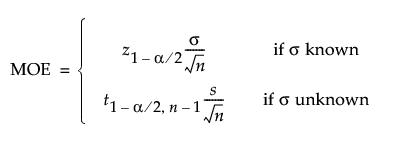The bound is calculated as follows: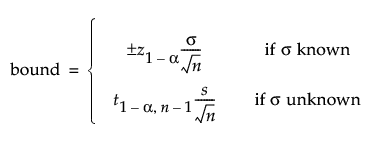#### Prediction Intervals

The margin of error (MOE) for prediction intervals is calculated as follows: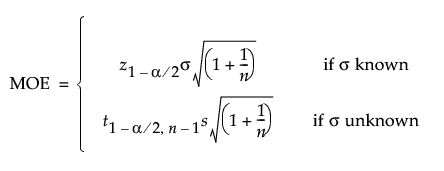The bound is calculated as follows: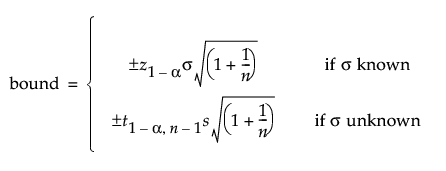#### Tolerance Intervals

For the tolerance interval on a proportion q of the population, the margin of error or bound is computed based on approximate procedures described in Krishnamoorthy and Mathew (2009).

The margin of error (MOE) for tolerance intervals is calculated as follows: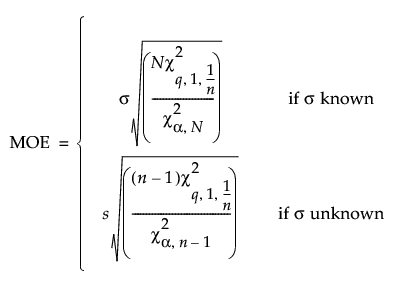The bound is calculated as follows: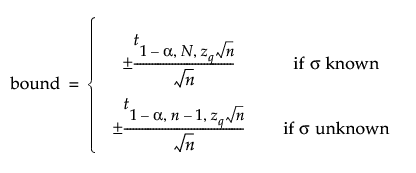where N is a suitably large integer value to represent asymptotic behavior. JMP sets the value of N to 2000.